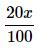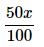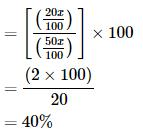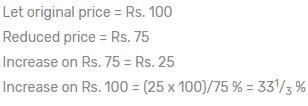Courses

# Percentage MCQ 1

## 20 Questions MCQ Test Quantitative Techniques for CLAT | Percentage MCQ 1

Description
This mock test of Percentage MCQ 1 for Quant helps you for every Quant entrance exam. This contains 20 Multiple Choice Questions for Quant Percentage MCQ 1 (mcq) to study with solutions a complete question bank. The solved questions answers in this Percentage MCQ 1 quiz give you a good mix of easy questions and tough questions. Quant students definitely take this Percentage MCQ 1 exercise for a better result in the exam. You can find other Percentage MCQ 1 extra questions, long questions & short questions for Quant on EduRev as well by searching above.
QUESTION: 1

Solution:
QUESTION: 2

### If two numbers are respectively 20% and 50% of a 3rd number then what % is the 1st number of the 2nd one?

Solution:

Let third number is x.

Then first no. 20% of x=Second number = 50% of x =Percent of first number of second number,QUESTION: 3

### If the radius of circle is increased by 110% its area is increased by

Solution:
QUESTION: 4

In an election between two candidates, one got 55% of the total valid votes, 20% of the votes were invalid. If the total number of votes was 7500, the number of valid votes that the other candidate got, was:

Solution:
QUESTION: 5

In an exam 80% of the students passed in Eng, 85% in Math and 75% in both. if 40 students failed in both subjects, the total number of students is?

Solution:

Let n(A) = no. of students pass in english
n(B) = no. of students pass in math
n(A U B) = no. of students pass in either math or english
n(A ∩ B) = no.of students pass in both math and english
let x are no. of students
⇒ n(A U B) = n(A) + n(B) - n(A ∩ B)
= 80x/100 + 85x/100 - 75x/100
= 90x/100
no. of students fail in both either math or english = x - n(A U B)

⇒ 40 = x - 90x/100

⇒ 40 =  x/10

⇒  x = 400
total no. of students = 400

QUESTION: 6

Mrs. Ruhani travels with 80% of her usual speed and thus gets late by 40 minutes. What will be the usual time taken by her in covering the same distances?

Solution:
QUESTION: 7

The price of rice is increased by 25% and as a result, a person can have 4kg less rice for Rs.400. What is the increase price of rice per kg?

Solution:
QUESTION: 8

In an examination, Summit got 30% marks and failed by 15 marks. In the same examination, Rohit got 40% marks, which is 35 marks more than the pass marks. Then what is the total marks in the examination?

Solution:
QUESTION: 9

After spending 40% in machinery, 25% in building, 15% in raw material and 5% in furniture, Harilal had a balance of Rs. 1305. The money with him was

Solution:
QUESTION: 10

In Mathematics exam, a student scored 30% marks in the first paper, out of the total of 180. How much should he score in second paper out of a total of 150, if he is to get an overall average of at least 50%

Solution:
QUESTION: 11

In an examination, it is required to get 36% of maximum marks to pass. A student got 113 marks and was declared fail by 85 marks. The maximum marks are

Solution:
QUESTION: 12

In an examination, 42% students failed in Hindi and 52% failed in English. If 17% failed in both the subjects, the percentage of those who passed in both the subject is

Solution: It is given that 42% fail in Hindi and 52% fail in English and 17% fail in both
So total fail percentage would be
42+52-17 ( because we have counted the 17 % 2 times)
=> 77 % who have failed SO the percentage passing in both would be
100-77= 23%
QUESTION: 13

In a college election, a candidate secured 62% of the votes and is elected by a majority of 144 votes. The total number of votes polled is

Solution:
∵ (62% of N - 38% of N) =144
⇒ 24% of N = 144
∴ N = (144 x 100)/24 = 600
QUESTION: 14

The price of sugar is increased by 20%. If the expenditure is not allowed to be increased, the ratio between the reduction in consumption and the original consumption is

Solution:
QUESTION: 15

On decreasing the price of TV set by 30%, its sale is increased by 20%. What is the effect on the revenue received by the shopkeeper?

Solution:
Lets take the orignal price as 100. Then on decreasing the price by 30% we get= 70. Now the sale is increased by 20%=84 (20% of 70 or 20/100*70= 14 now 70+14=84) So we can say that Cp=100 and Sp=84 Thats loss as cp is bigger. Loss=16 Loss%=16/100*100 =16%

QUESTION: 16

The value of a machine depreciates 10% annually. If its present value is Rs. 4000, its value 2 years hence will be:

Solution:
QUESTION: 17

The population of a town increases by 5% annually. If it is 15435 now, its population 2 years ago was:

Solution:
QUESTION: 18

A man’s wages were decreased by 50%. Again the reduced wages were increased by 50%. He has a loss of:

Solution:
QUESTION: 19

A number when increased by 37 ½% gives 33. The number is:

Solution:
QUESTION: 20

The price of an article has been reduced to 25%. In order to restore the original price, the new price must be increased by:

Solution: